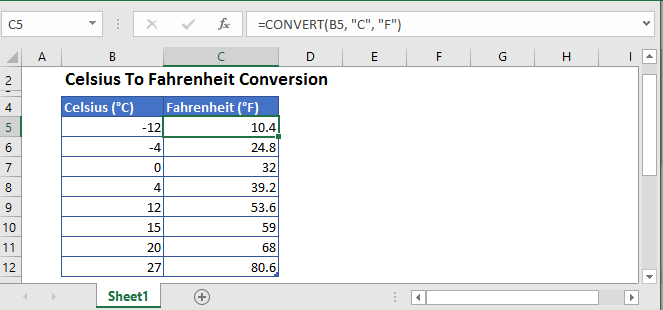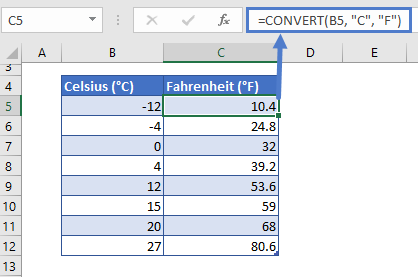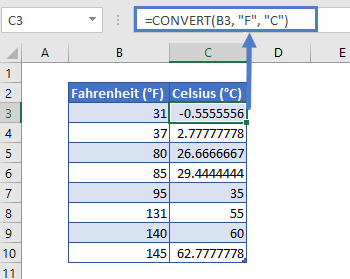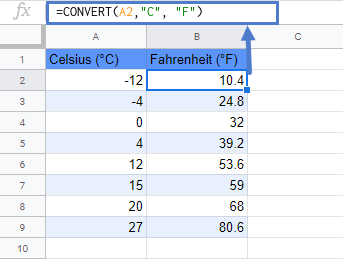# Convert Celsius to Fahrenheit in Excel & Google Sheets

This tutorial will demonstrate how to convert temperatures from Celsius to Fahrenheit in Excel & Google Sheets.## Conversion of Celsius to Fahrenheit

The CONVERT function can be used to convert temperature from Celsius to Fahrenheit.

Let’s say in column B we have temperatures recorded as Celsius and in column C we want the equivalent value in Fahrenheit. Type the following formula in cell C5 and drag it down:

``=CONVERT(B5, "C", "F")``The CONVERT function converts a number from one measurement unit to another.

And for conversion, the CONVERT function depends on the unit from which conversion is taking place and to the required unit of the measurement.

## Conversion of Fahrenheit to Celsius

If you want to convert temperature from Fahrenheit to Celsius, just use the following formula:

``=CONVERT(B3, "F", "C")``## Conversion from Celsius to Fahrenheit in Google Sheets

The conversion of Celsius to Fahrenheit formula works exactly the same in Google Sheets as in Excel:### Excel Practice Worksheet

Practice Excel functions and formulas with our 100% free practice worksheets!